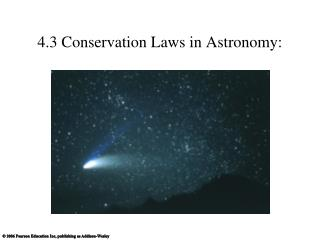DownloadDownload Presentation4.3 Conservation Laws in Astronomy:

# 4.3 Conservation Laws in Astronomy:

Télécharger la présentation## 4.3 Conservation Laws in Astronomy:

- - - - - - - - - - - - - - - - - - - - - - - - - - - E N D - - - - - - - - - - - - - - - - - - - - - - - - - - -
##### Presentation Transcript

1. 4.3 Conservation Laws in Astronomy:

2. Momentum • Momentum: a property of moving objects • Momentum = mass  velocity • Formula, P = m v • A net force changes the momentum of an object

3. Conservation of Momentum • The total momentum of a system can’t change unless an external force is applied • Interacting objects exchange momentum through equal and opposite forces

4. Spinning or orbiting objects have angular momentum • Angular momentum = mass x velocity x radius L = m v r

5. Conservation of Angular Momentum • The angular momentum of an object can’t change unless an external twisting force is applied • Earth experiences no twisting force as it orbits the Sun, so its rotation and orbit will continue indefinitely

6. Conservation of angular momentum explains why objectsrotate faster as they shrink in radius: m x v x R = m x V x r

7. For an orbiting body:

8. Energy makes matter move.

9. Potential Energy: Gravitational GPE depends on: • object’s mass (m) • Acceleration due to gravity (g) • distance object could potentially fall

10. GPE • In space, an object or gas cloud has more gravitational energy when it is spread out than when it contracts. • A contracting cloud converts gravitational potential energy to thermal energy.

11. KE & GPE for an orbiting body:

12. Kinetic Energy: Thermal • Thermal energy is the total kinetic energy of many particles (for ex in air, water, rock) • Related to temperature but it is NOT the same • Temperature is the average kinetic energy of the many particles in a substance.

13. Mass itself is a form of potential energy • A small amount of mass can release a great deal of energy • E = mc2

14. Conservation of Energy • Energy cannot be created or destroyed. • It can change form or be exchanged between objects. • The total energy content of the Universe was determined in the Big Bang and remains the same today.